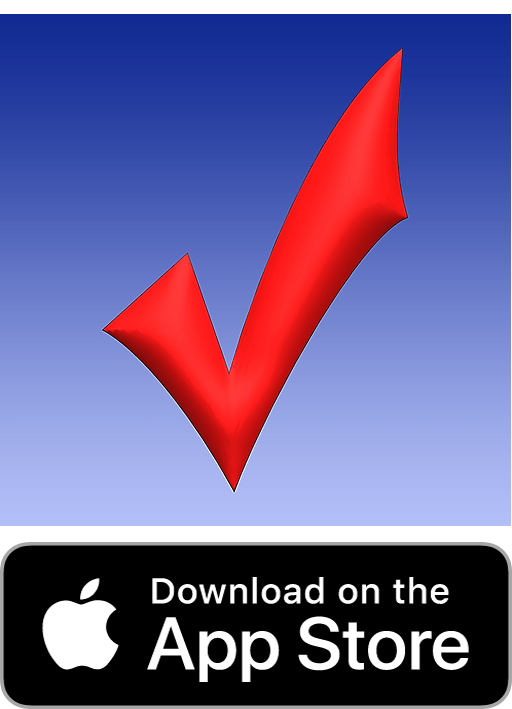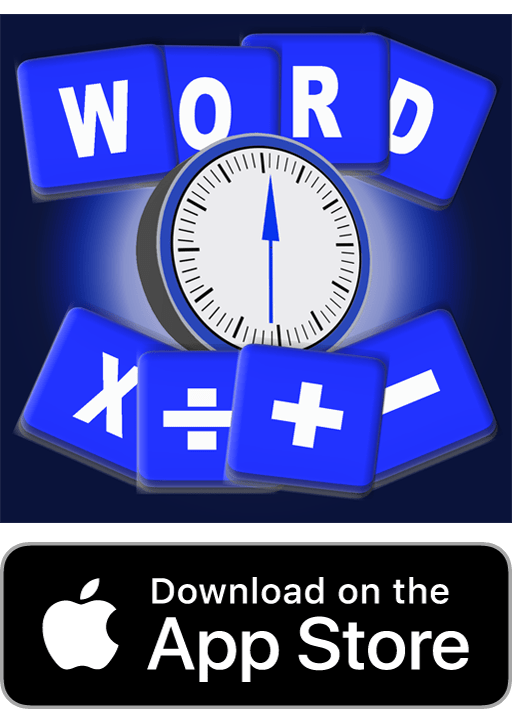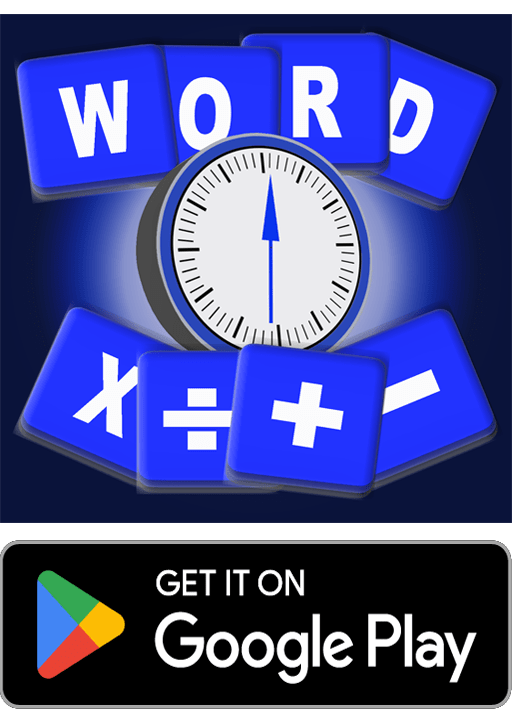## How to improve mental agility

How to improve mental agility is something a lot of us would like to do. Obviously an important part of mental maths is knowing the multiplication tables. Consequently train your brain to respond quickly. As Spencer Greenberg a mathematician and founder of ClearerThinking.org says memorising these “basic building blocks” of maths, enables the instant finding of answers to simple problems which are part of more difficult ones. Therefore revise your tables and not just the basic ones. Learn the 13 times table, 25 times table (easy peasy), 75 times table (recommended by Lily from Letters and Numbers Show on SBS) and 125 times table. Surprisingly we we were taught that at Primary School in the Stone Age.

125, 250, 375, 500, 625, 750, 875, 1000

Our Spelling App available in the Apple Store not only lets you practise your school spelling lists, it provides you with practice for your times tables including the thirteen, 25 and 125 times tables.## How to improve mental agility Other strategies

Obviously memory is very important and memorising some basic calculations will speed up your thinking.

### Number I divisions

Memorise as well 1 divided by various numbers.

a)  1/2 =0.5     b)  1/3 = 0.333    c)  1/4 = 0.25   d)   1/5 = 0.2   e)  1/6 = 0.166

### Multiplication Tips

One obvious rule is when multiplying a number by 10, it just needs to have a zero added. When multiplying by 5 the answer must end in a 0 or a 5.

When multiplying by 12, multiply by 10, and then by 2 and add the two numbers together.

When multiplying by 15, multiply by 10 and then add half the answer eg 4 x 15 = 4 x 10 = 40 plus half the answer, 20 making 60 in total.

There is a trick to multiplying by 16. Multiply by 10 first, then multiply half the number by 10. Add the two together plus the number itself.

eg     i)16 x 22;     ii)10 x 22 = 220,     iii)10 x 11 = 110;      iv)220+110+22 =352.

You can help your children by doing some of these multiplications with them. AppMum’s Handy Homework will give lots of practice opportunities for parents and children and maths can be fun! Give it a go!

Another way to practise number skills it to play our Letters and Numbers App. It is available for free download from the Apple Store. Click on the icon below.